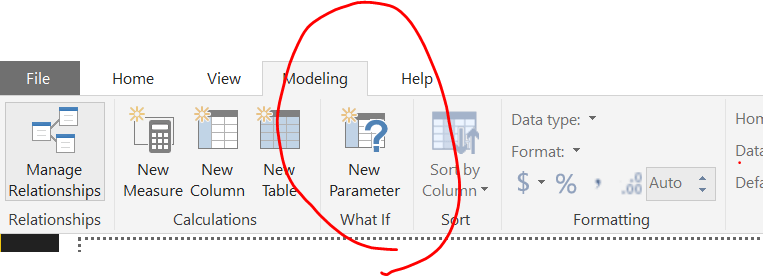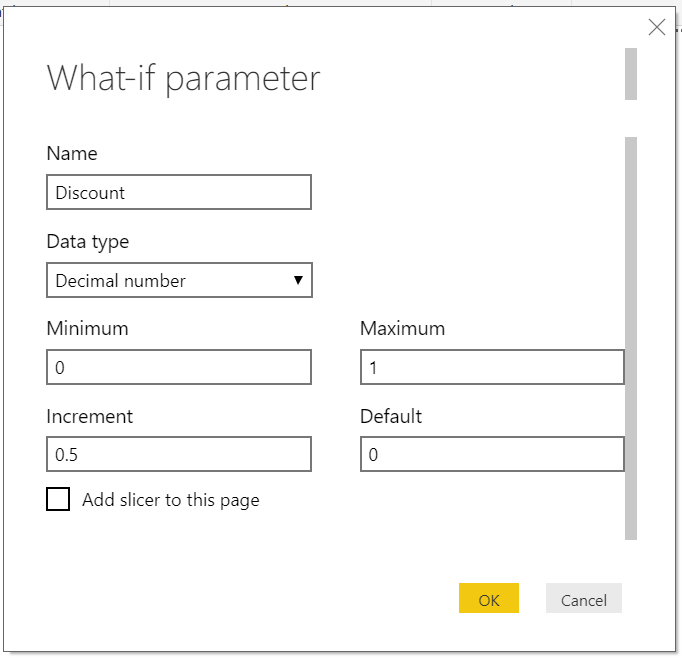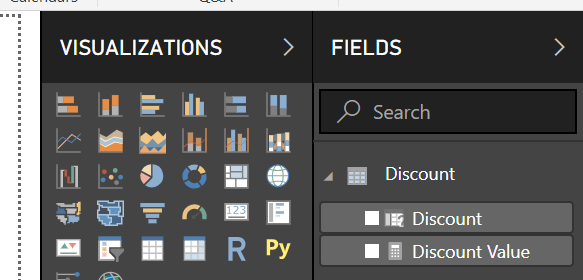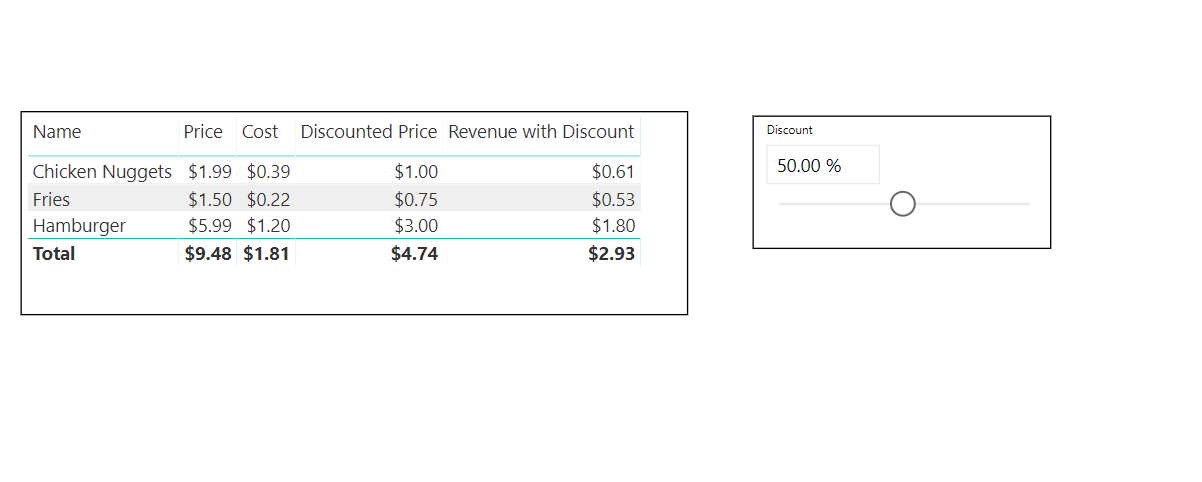# What If Parameter in Power BI

Using What If parameter in Power Bi can easily give you the ability to dynamically transform your data. For example, using this parameter will allow to demonstrates how your data change under various scenarios. For example how much revenue would you have if your products were at 10%, 20% or 30%  of the retail price. You could add additional logic to show how this changes demand. Another scenario would be to show create a marketing mix to show how profit would change due to different investment in each channel.How to use What IF parameters in Power BI.

1. Click the Modeling tab  in the top ribbon.
2. Click the What IF parameter from the top ribbon.
3. The What If parameter window will open
4. Provide details such as Name, Data Type, Minimum, Maximum and Default number.
5. Lastly, you can add an optional slicer.
6. A table with a calculated measure will be created
• A generated series that spans the specifications of your parameter
• A selected value function that changes as the parameter changes.

Let’s see this in action

Scenario: You have a list of sales items with name, price, and cost. I would like to create a parameter that allows me to apply discounts so that I can see the overall revenue of each item when different discounts are applied.  I would like to have a parameter that spans from 0% to 100% with a 5% increment.You can see how this parameter is created by viewing the new table:Once the What If parameter is created you have your generated series that looks like:

Discount = GENERATESERIES(0, 1, 0.5)

And you will have a selected value functions that looks like this:

Discount Value = SELECTEDVALUE(‘Discount'[Discount], 0)

Both of these are automatically created for you.

Apply the parameter to your data

In this case, I want to apply the discount to my price. This can be easily done with a calculated measure. As below you see below, I simply multiply the price by my discount value.

Discounted Price = Discount[Discount Value]* SUM(Items[Price])

The final result you can pull into a table so that you see how the price is affected by the discount parameter as you slide it to different discount values.#### Gaelim Holland

Subscribe
Notify of1 Comment
Inline Feedbacks